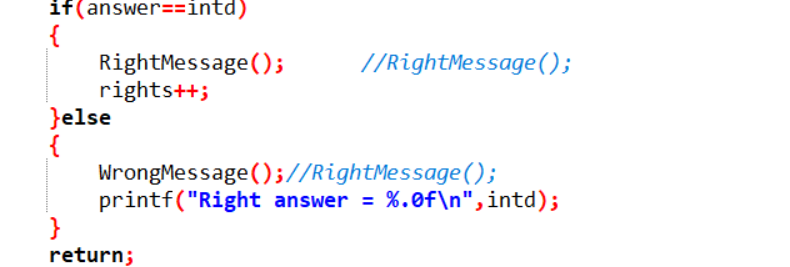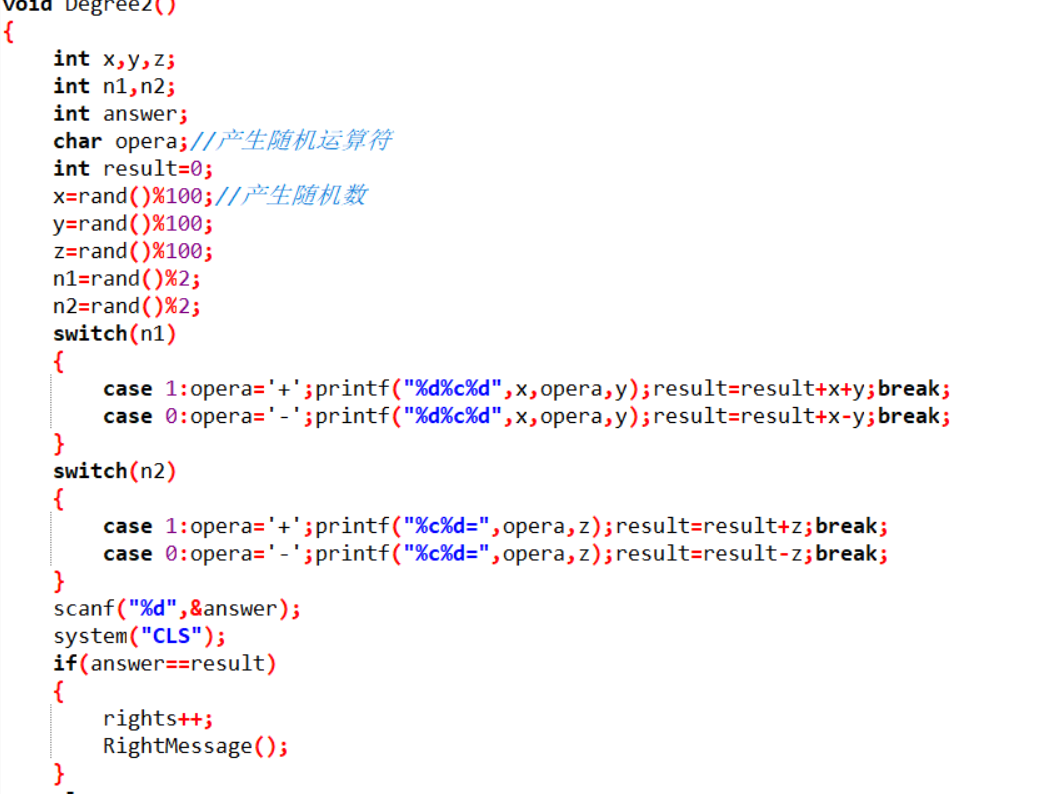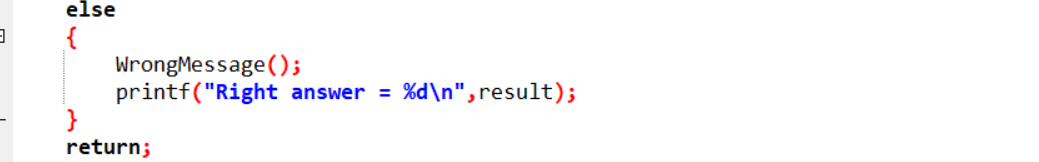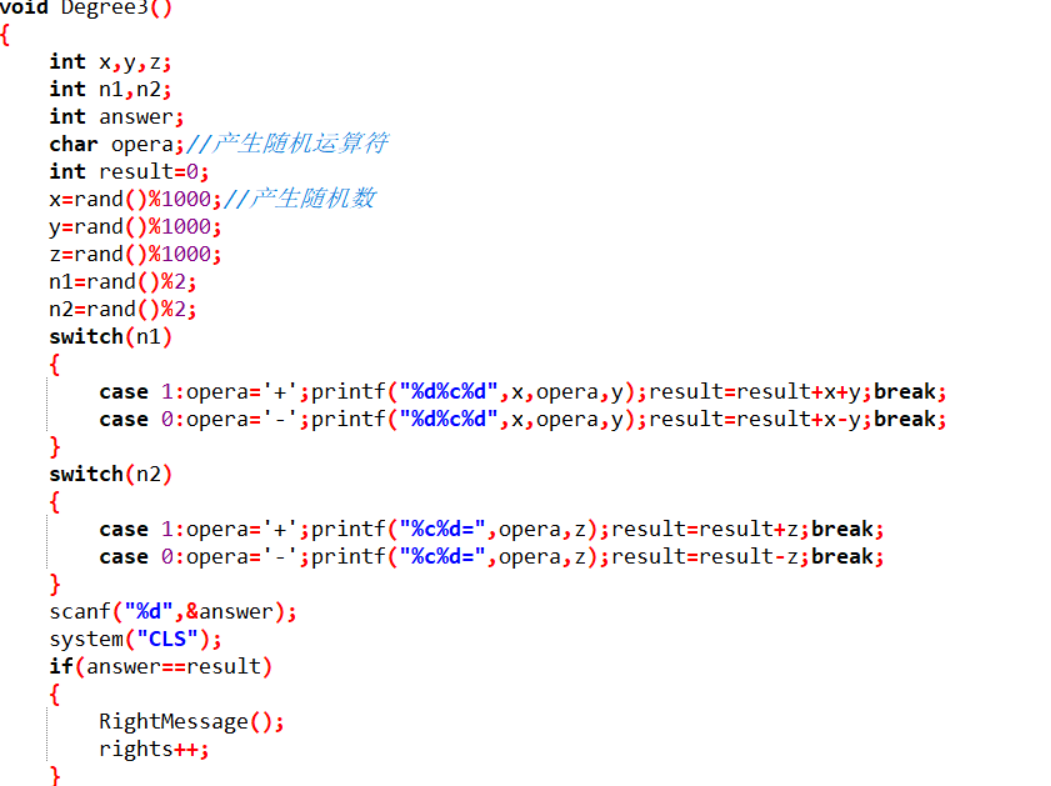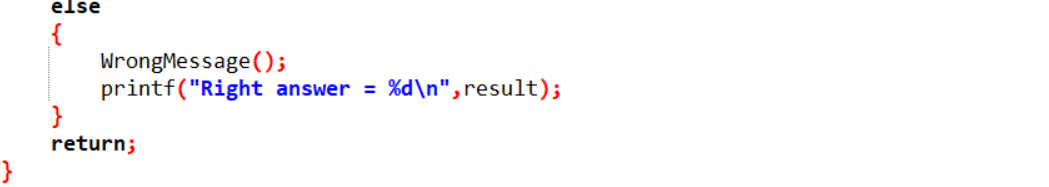# 1.本章学习总结

## 1.1思维导图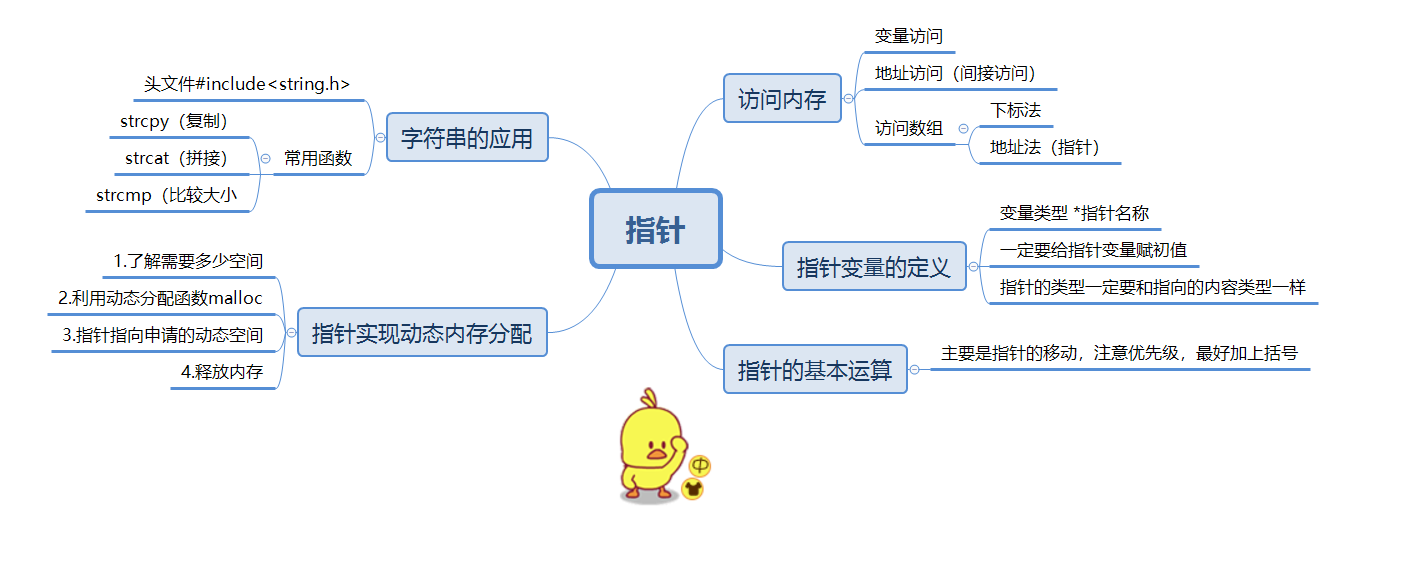## 1.2 本章学习体会及代码量学习体会

### 1.2.2代码累计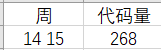# PTA总分

## 2.1排名截图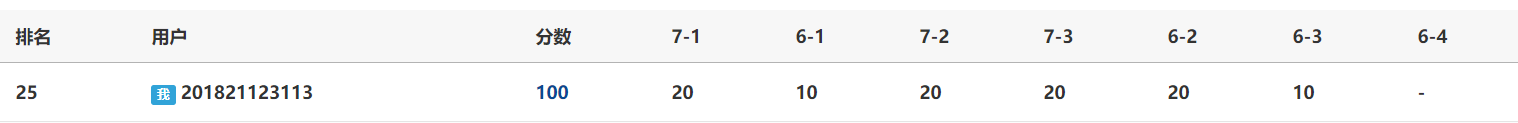# PTA作业

## 3.1

7-3 字符串的冒泡排序 （20 分）

## 3.1.1算法分析

char a
for i=0 to n;
scanf("%s",a[i]);//输入字符串
end for
static char temp//定义临时数组，用于交换
for j=1 to k
for j=1 to k
if a[i+1]<a[i]

end if
end for
end for
for i=0 to n

end for

### 3.1.2代码截图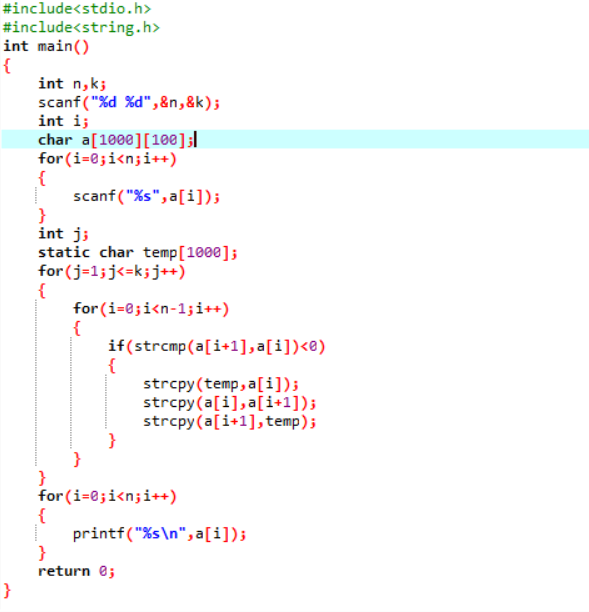### 3.1.3数据测试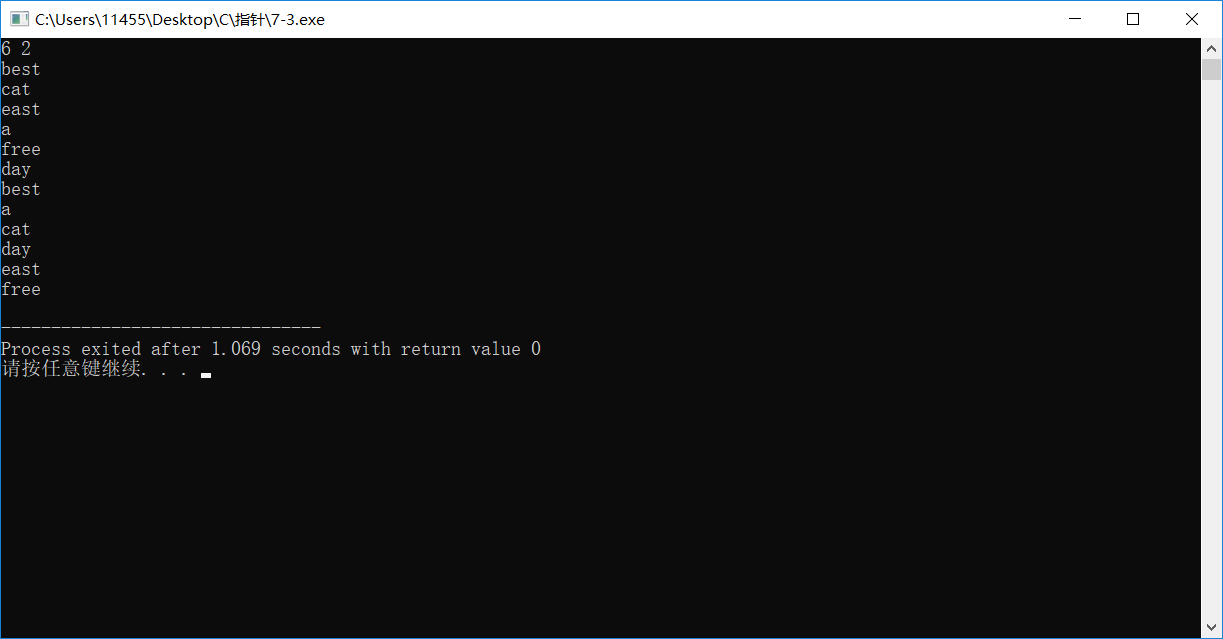### 3.1.4提交列表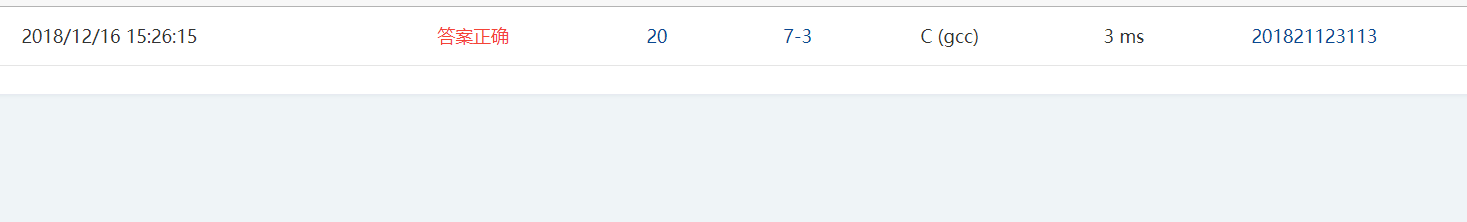int n,k

# 4大作业

## 4.1改造函数介绍

### 4.1.1函数1——生成算式并计算结果

void Question(char degree)//把上一级选择难度函数的选择级别传入
{
char ques//放算式
int level = degree-'0'//将难度级别的字符转换成常数
int m,n//n为运算符个数
char a//把数转换成字符串
switch level do
case 1 do n=4;i=3;break;
case 2 do n=2;i=5;break;
case 3 do n=2;i=5;break;
end switch
for i to i>0 j=0,a
m=rand()%n;
if i为奇数
switch m do
m=rand()%4;
1,2,3,4分别代表+,-,*,/ //strcat(ques,"+")连接字符串
end switch
else i为偶数
swtich level do
case 1 do 生成一位数,break;//生成的数放在x[j]中
case 2 do 生成二位数,break;
case 3 do 生成三位数,break;
end switch
if语句排除除法结果不存在和得到小数的情况
itoa(x[j],a,10);
strcat(ques,a)
end if
j++;
end for

##### 代码截图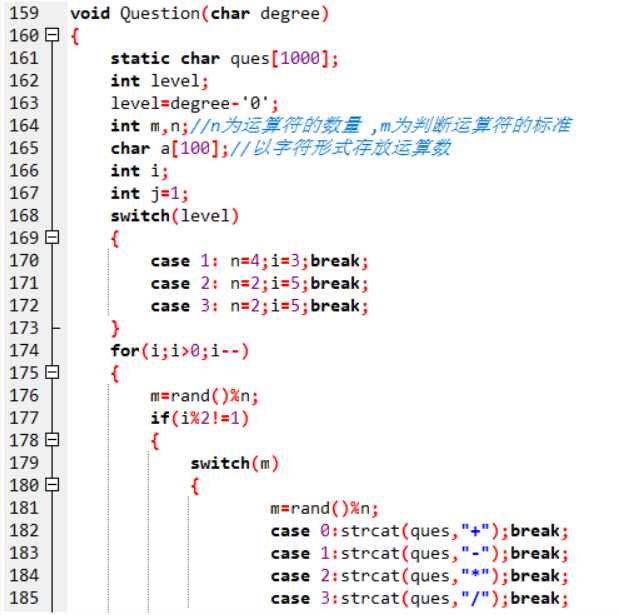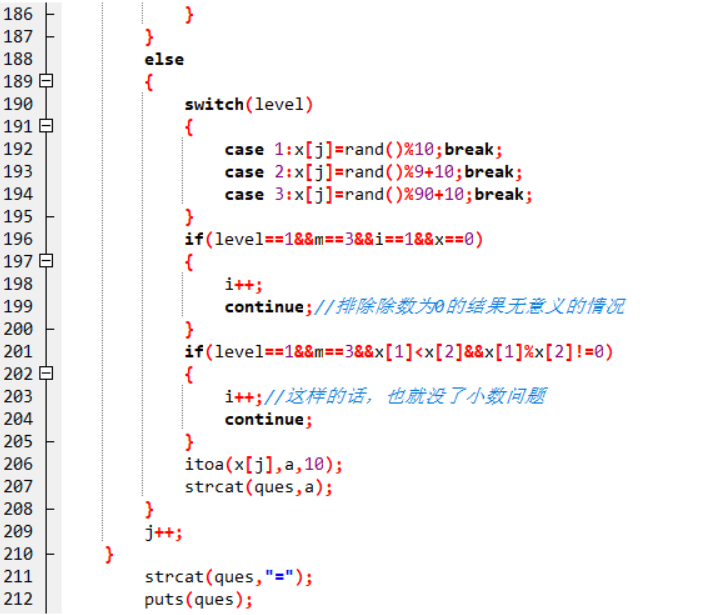### 查看成绩函数

#### 代码截图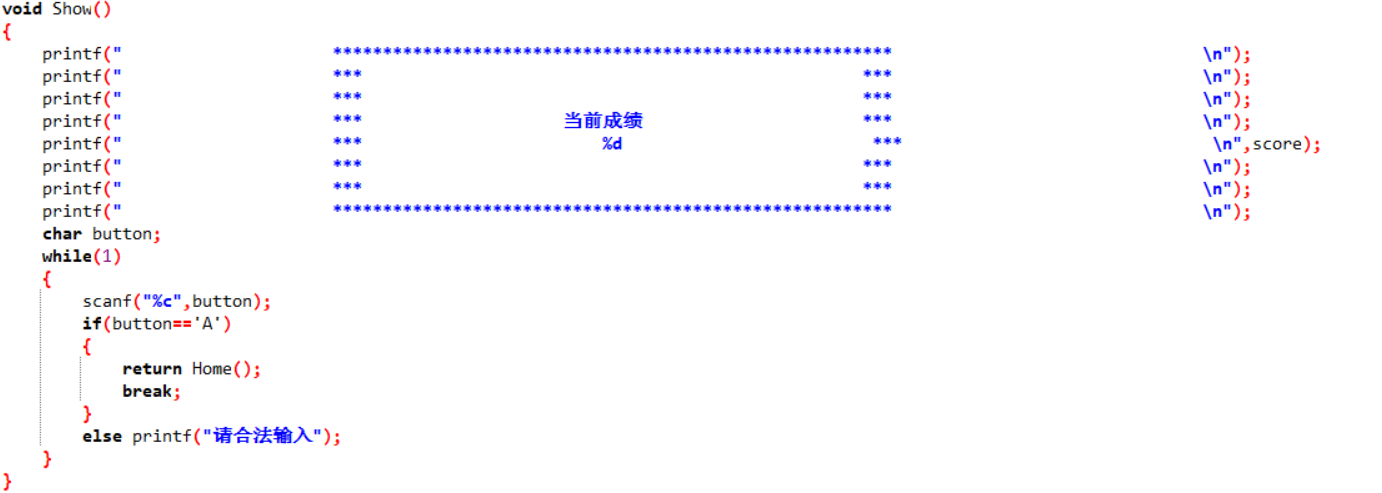## 代码比较

### 函数1